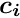Millepede-II  V04-08-02
Major changes

Major changes with respect to the draft manual.

# Solution methods

The following methods to obtain the solutionfrom a linear equation systemare implemented:

## Inversion

The solution and the covariance matrixare obtained by inversion of. Available are the value, error and global correlation for all global parameters. The matrix inversion routine has been parallelized and can be used for up to several 10000 parameters.

## Diagonalization

The solution and the covariance matrixare obtained by diagonalization of. Available are the value, error, global correlation and eigenvalue (and eigenvector) for all global parameters.

## Minimal Residual Method (MINRES)

The solution is obtained by minimizingiteratively. MINRES [ref 8] is a special case of the generalized minimal residual method (GMRES) for symmetric matrices. Preconditioning with a band matrix of zero or finite bandwidth is possible. Individual columnsof the covariance matrix can be calculated by solvingwhereis the i-th column on the unit matrix. The most time consuming part (product matrix times vector per iteration) has been parallelized. Available are the value for all (and optionally error, global correlation for few) global parameters.

## Advanced Minimal Residual Method (MINRES-QLP)

The MINRES-QLP implementation [ref 9] is a MINRES evolution with improved norm estimates and stopping conditions (leading potentially to different numbers of internal iterations). Internally it uses QLP instead of the QR factorization in MINRES which should be numerically superior and allows to find for singular systems the minimal length (pseudo-inverse) solution.

The default behavior is to start (the internal iterations) with QR factorization and to switch to QLP if the (estimated) matrix condition exceeds mrtcnd. Pure QR or QLP factorization can be enforced by mrmode.

## Elimination of constraints

As alternative to the Lagrange multiplier method the solution by elimination has been added for problems with linear equality constraints. A QL factorization (with Householder reflections) of the transposed constraints matrix is used to transform to an unconstrained problem. For sparse matrix storage the sparsity of the global matrix is preserved.

## Decomposition

The solution is obtained by a root-free Cholesky decomposition (LDLt). The covarinance matrix is not being calclulated. The method ia about a factor 2-3 faster than inversion (according to several tests). It is restricted to solution by elimination for problems with linear equality constraints (requires positive definite matrix).

# Regularization

Optionally a termcan be added to the objective function (to be minimized) whereis the vector of global parameters weighted with the inverse of their individual pre-sigma values.

# Local fit

In case the local fit is a track fit with proper description of multiple scattering in the detector material additional local parameters have to be introduced for each scatterer and solution by inversion can get time consuming (~forlocal parameters). For trajectories based on broken lines [ref 4,6] the corresponding matrixhas a bordered band structure (for(border size) and(bandwidth)). With root-free Cholesky decomposition the time for the solution is linear and for the calculation of(needed for the construction of the global matrix) quadratic in. For each local fit the structure ofis checked and the faster solution method selected automatically.

# Parallelization

The code has been largely parallelized using OpenMP™. This includes the reading of binary files, the local fits, the construction of the sparsity structure and filling of the global matrix and the global fit (except by diagonalization). The number of threads is set by the command threads.

Caching. The records are read in blocks into a read cache and processed from there in parallel, each record by a single thread. For the filling of the global matrix the (zero-compressed) update matrices (from equations (15), (16)) produced by each local fit are collected in a write cache. After processing the block of records this is used to update the global matrix in parallel, each row by a single thread. The total cache size can be changed by the command cache.

# Sparse matrix storage

## Compression

In sparse storage mode for each row the list of column indices (and values) for the non-zero elements are stored. With compression regions of continous column indices are represented by the first index and their number (packed into a single 32bit integer). Compression is selected by the command compress. In addition rare elements can be neglected (,histogrammed) or stored in single instead of double precision according to the pairentries command.

## Parameter groups

With the implementation of parameter groups (sets of adjacent global parameters (labels) appearing in the binary files always together) the sparsity structure addresses not single values anymore but block matrices with sizes according to the contributing parameter groups. Groups with adjacent column ranges are combined into a single block matrix. The offset and first column index in a (block) row is stored.

# Gzipped C binary files

The zlib can be used to directly read gzipped C binary files. In this case reading with multiple threads (each file by single thread) can speed up the decompression.

# Transformation from FORTRAN77 to Fortran90

The Millepede source code has been formally transformed from fixed form FORTRAN77 to free form Fortran90 (using TO_F90 by Alan Miller) and (most parts) modernized:

• IMPLICIT NONE everywhere. Unused variables removed.
• COMMON blocks replaced by MODULEs.
• Backward GOTOs replaced by proper DO loops.
• INTENT (input/output) of arguments described.
• Code documented with doxygen.

Unused parts of the code (like the interactive mode) have been removed. The reference compiler for the Fortran90 version is gcc-4.6.2 (gcc-4.4.4 works too).

# Memory management

The memory management for dynamic data structures (matrices, vectors, ..) has been changed from a subdivided static COMMON block to dynamic (ALLOCATABLE) Fortran90 arrays. One Pede executable is now sufficient for all application sizes.